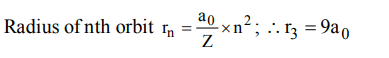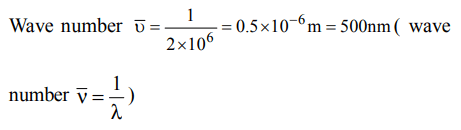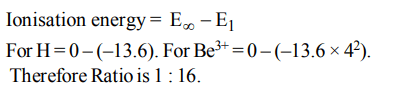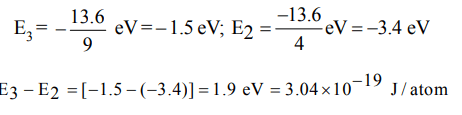## Atomic Structure Questions and Answers Part-7

1. The energy of an electron in the first Bohr orbit of H atom is – 13.6 eV. The possible energy value(s) of the excited state (s) for electrons in Bohr orbits of hydrogen is (are)
a) – 3.4eV
b) – 4.2eV
c) – 6.8 eV
d) + 6.8eV

Explanation:2. If the radius of first Bohr orbit be $a_{0}$ , then the radius of the third orbit would be
a) $3 ×a_{0}$
b) $6 ×a_{0}$
c) $9 ×a_{0}$
d) $1/9 ×a_{0}$

Explanation:3. According to Bohr’s theory of hydrogen atom, which of the following is quantised for an electron?
a) Angular momentum
b) Angular acceleration
c) Acceleration
d) Velocity

Explanation: Angular momentum is quantised

4. According to Bohr’s theory the energy required for the transition of H-atom from n = 6 to n = 8 state is
a) equal to the energy required for the transition from n = 5 to n = 6 state
b) larger than in (a)
c) less than in (a)
d) equal to the energy required for the transition from n = 7 to n = 9 state.

Explanation: The difference between the energy of adjacent energy levels decreases on moving away from nucleus

5. The wave number of the light emitted by a certain source is $2 ×10^{6}$ . The wavelength of this light is
a) 500 m
b) 200 nm
c) 500 nm
d) $5 ×10^{7}$

Explanation:6. For which of the following species, Bohr’s theory is not applicable?
a) $Be^{3+}$
b) $Li^{2+}$
c) $He^{2+}$
d) H

Explanation: He2+ does not contain any electron. Bohr's theory is applicalbe to species containing one electron.

7. As the nuclear charge increases from Neon to Calcium, the orbital energies
a) increase
b) increase very rapidly
c) increase very slowly
d) fall

Explanation: En $\propto$ Z2 increases rapidly

8. The ratio of ionization energy of H and $Be^{+3}$ is
a) 1 : 1
b) 1 : 3
c) 1 : 9
d) 1 : 16

Explanation:9. In a Bohr model of on atom, when an electron jumps from n = 3 to n = 1, how much energy will be emitted?
a) $2.15 × 10^{-11}ergs$
b) $2.389 × 10^{-12}ergs$
c) $0.239 × 10^{-10}ergs$
d) $0.1936 × 10^{-10}ergs$a) $3.03 × 10^{-19}J/atom$
b) $1.03 × 10^{-19}J/atom$
c) $3.03 × 10^{-12}J/atom$
d) $6.06 × 10^{-19}J/atom$